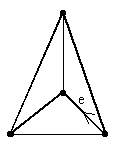## Project 4: Hamiltonian Circuits Arising from Coded Paths in 3-valent Plane Graphs

Let G be a plane 3-valent graph and e be one of its edges. Suppose that by moving along some edge one can generate a circuit which:

a. Closes into a simple closed curve.

b. Passes through each vertex of the graph once and only once (i.e. Hamiltonian circuit) by following a "coded sequence" over and over again.

Examples of coded sequences are: L, R, L, R, .... and L, L, R, R, L, L, R, R, .... where L and R refer to making left and right turns, respectively, when one gets to the vertex at the end of an edge.

For example, Figure 1 shows an example of a graph where this occurs for the the L, R code. The edge e is shown and an arrow indicates the direction of motion along e. The dark edges are those that are generated by applying the L, R code.Figure 1

Problems

1. Determine which 3-valent plane graphs have hamiltonian circuits generated by the L,R code.

2. Determine which 3-valent plane graphs have hamiltonian circuits generated by the L,L,R,R code; by the L,R,R,L code.

Extensions

1. Extend your results to graphs which have valence other than 3.

References:

1. Ore, O., Graphs and their Uses, Mathematical Association of America, Washington, 1963. (There is a new revised edition prepared by R. Wilson.)

2. West, D., Graph Theory, Prentice-Hall, Englewood Cliffs, 1996.

Joseph Malkevitch
Department of Mathematics and Computing
York College (CUNY)
Jamaica, New York 11451-0001
email: malkevitch@york.cuny.edu
(Comments and results related to the project above are welcome.)

Acknowledgements
Some of this work was prepared with partial support from the National Science Foundation (Grant Number: DUE 9555401) to the Long Island Consortium for Interconnected Learning (administered by SUNY at Stony Brook, Alan Tucker, Project Director).